Log Overlays and Crossplots to Quantify Fractures
Quantitative fracture methods include fracture intensity calculations that help to discriminate between lightly fractured and heavily fractured intervals. Fracture porosity and fracture permeability are covered as well as secondary porosity index and Pickett plots for finding the cementation exponent, M.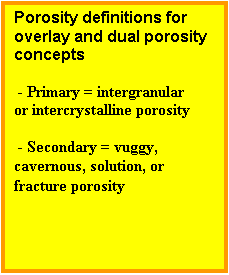Sonic/density or sonic/neutron porosity overlay presentations help find vugs and caverns in carbonates. Fractures are often associated with these porosity types. Sonic derived porosity is generally considered to be intergranular or intercrystalline (primary) porosity, whereas density or neutron derived porosity measures primary (intergranular or intercrystalline) plus secondary (vuggy, solution, or fracture) porosity. Note that the words primary and secondary porosity are used here in their traditional log analysis sense and not in a strict geological sense. However, much of the log analysis literature, especially with respect to the dual porosity model for fracture analysis, uses the terms as described in this paragraph. As mentioned earlier, fracture porosity is very small and is usually overwhelmed by the vuggy portion.

Density neutron crossplot porosity minus sonic porosity yields a result, traditionally called secondary porosity index or SPI, usually attributed to vugs or caverns, and to a lesser degree, fractures.
1. SPI = PHIsec = Max(0, PHIxnd – PHIsc)

Where:
SPI = PHIsec = secondary porosity index
PHIxnd = density neutron crossplot porosity corrected for shale and lithology
PHIsc = sonic porosity corrected for shale and lithology

The calculation rules for PHIxnd and PHIsc are defined elsewhere in this Handbook. Raw logs seldom have the correct scales to make an adequate overlay, so computer processed curves are usually used. Lithology must be known or computed accurately for this comparison to be valid; this is possible in pure limestone sections but not always in mixed lithology.

If fracturing is sufficient enough to increase the total porosity substantially, the porosity comparison method allows fractured zones to be detected. This is usually not the case, but if an increase in porosity of more than 1 or 2% due to fracturing is present, it certainly can be seen.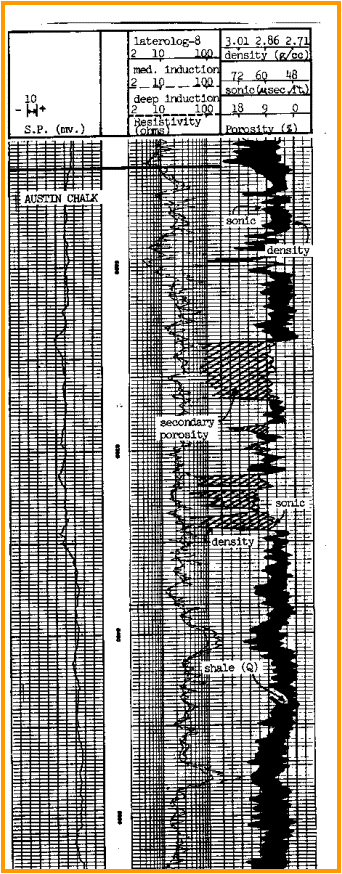This illustration shows an Austin Chalk example. The cross hatched area on the log defines zones where density porosity is greater than sonic porosity. In this case, it looks like the difference is due to rough or large borehole, and not entirely to fracture porosity. However, the presence of fractures is almost certain.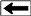Density - sonic overlay in Austin Chalk

A better plot would use the neutron or density neutron crossplot porosity compared to the sonic porosity, with the sonic porosity computed with a matrix travel time derived from the density neutron or density neutron PE lithology calculation. The black shading shows intervals where density porosity is lower than sonic. The example claims this is due to shaliness, but some of it may be due to inadequate lithology compensation in the sonic porosity calculations.

Methods have been developed using the above porosity measurements which lend themselves better to computer analysis. For example, by crossplotting Mlith and Nlith values, points which fall in certain areas of the crossplot could represent secondary porosity, ie. vuggy porosity plus fracture porosity. Secondary porosity raises the Mlith value compared to the same rock with no secondary porosity.

Other crossplots using porosity from sonic, neutron, or density versus each other or gamma ray, and matrix density versus matrix travel time (MID plot) are also used to solve particular cases. Crossplots are not as helpful as depth plot overlays.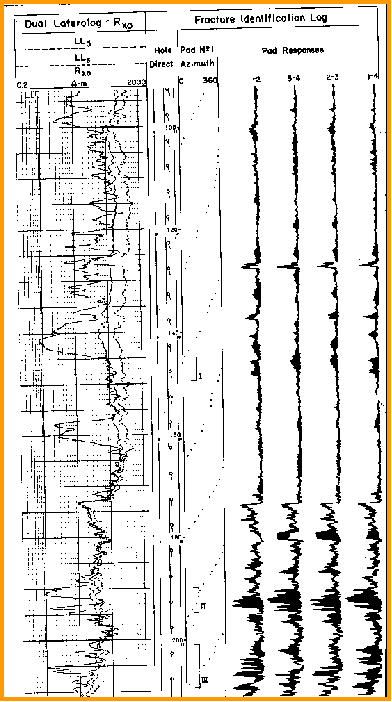A deep resistivity/Rxo overlay log is useful in spotting the shallow resistivity crossover caused by fractures. Rxo is the calculated value of the formation resistivity with the mud filtrate filling all pores. The value is derived from the shallowest resistivity device that was run. This might be a microlog or proximity log, which are often run on linear scales, making it difficult to compare to the deep resistivity on a logarithmic scale. Compatible scales are made in the computer truck or computer center so the analyst can see what is happening. When Rxo is less than the deep resistivity in fresh muds, vertical fractures are indicated.

Shallow resistivity overlay compared to dipmeter FIL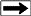Another approach is to make a crossplot, using logarithmic scales, of the apparent formation factor against porosity. The plot represents the Archie formation factor equation:
1. F = A / (PHIe ^ M) = Rxo / RMF@FT
= Ro / RW@FT

Where:
F = formation factor (unitless)
A = tortuosity constant (unitless)
M = cementation exponent (unitless)
PHIe = effective porosity (unitless)
Rxo = resistivity of invaded zone (ohm-m)
RMF@FT = mud filtrate resistivity at   formation temperature (ohm-m)
Ro = resistivity of un-invaded zone water zone (ohm-m)
RW@FT = formation water resistivity at formation temperature (ohm-m)

When the cementation exponent, M, is a constant it corresponds to a straight line of constant slope passing through the point F = A and PHIe = 1.0 on this plot. The tortuosity constant, A, is often taken equal to 1 for this analysis.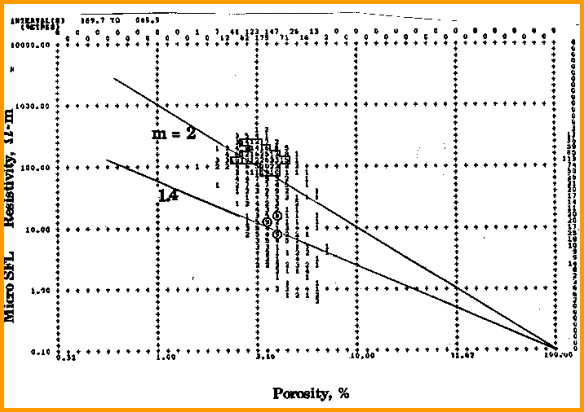Porosity - resistivity crossplot (Pickett plot) identifies fractures

In a non-fractured zone, the apparent M will be slightly too high if hydrocarbons are present. In a fractured zone, M will be much lower. Most of the points in this very tight zone (average porosity = 3%) plot above the M = 2.0 line due to residual gas saturation. Many points however plot at much lower values of M and range down to M = 1.1, with the predominate value near M = 1.4. Low values of M are common in fractured reservoirs. The more heavily fractured zones give the lowest M values.

Page Views ---- Since 01 Jan 2015
Copyright 2023 by Accessible Petrophysics Ltd.
CPH Logo, "CPH", "CPH Gold Member", "CPH Platinum Member", "Crain's Rules", "Meta/Log", "Computer-Ready-Math", "Petro/Fusion Scripts" are Trademarks of the Author